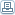# How Do Credit Card Interest Rates Work?

 By: Court TuttleCredit card interest is the principal way in which card issuers generate revenue. A card issuer is a bank that gives a consumer a card or account number that can be used with various payees to make payments and borrow money from the bank simultaneously. The bank pays the payee and then charges the cardholder interest over the time the money remains borrowed. Banks suffer losses when cardholders do not pay back the borrowed money as agreed. Typical credit cards have interest rates between 7 and 36 percent, depending upon the banks risk evaluation methods and the borrowers credit history. The cardholds credit risk is key to a card issuers profitability. Banks check national and international credit bureau reports that identify the borrowing history of the applicant. Different Methods For Charging Interest The Average Daily Balance is the simplest of the four methods, in the sense that it is an interest rate that produces approximately, if not exactly, equal to the expected rate. The sum is divided by the number of days covered in the cycle to give an average balance for that period. This amount is multiplied by a constant factor to give an interest charge. The result interest is the same as if interest was charged at the close of each day, except that it only compounds (added to the principal) once per month Next is the Adjusted Balance method where at the end of the billing cycle it is multiplied by a factor in order to give the interest charge. This can result in an actual interest rate lower or higher than the expected one, since it does not take into account the average daily balance. What matters here is the time the money was actually lent out by the bank. The longer the period the higher the interest rate because you are using their money, which increases their risk on you. The Previous Balance is the reverse of the Adjusted Balance. The balance at the start of the previous billing cycle is multiplied by the interest factor in order to derive the charge. As with the Adjusted Balance method, this method can result in an interest rate higher or lower than the expected one, but the part of the balance that carries over more than two full cycles is charged as the expected rate. Now let's take a look at the APR that is the principal means of comparing credit interest. It is compounded on a monthly basis. Most major banks use the following methodology: Increase the figure to the highest possible value while still meeting advertising requirements, e.g., if a card is advertised at a percentage rate of 17.9, then any value up to 17.949 will still be rounded down to 17.9. To derive the month rate, obtain the twelfth root. This will provide you will a rate which when compounded over a year will equal the APR. At this point, it is important to round down, since the APR has already been maximized. Pushing the APR up onto a higher rate could make the card issuer liable for false advertising claims. These are the four main methods banks, credit unions, etc; calculate their programs of charging interest for their credit cards.